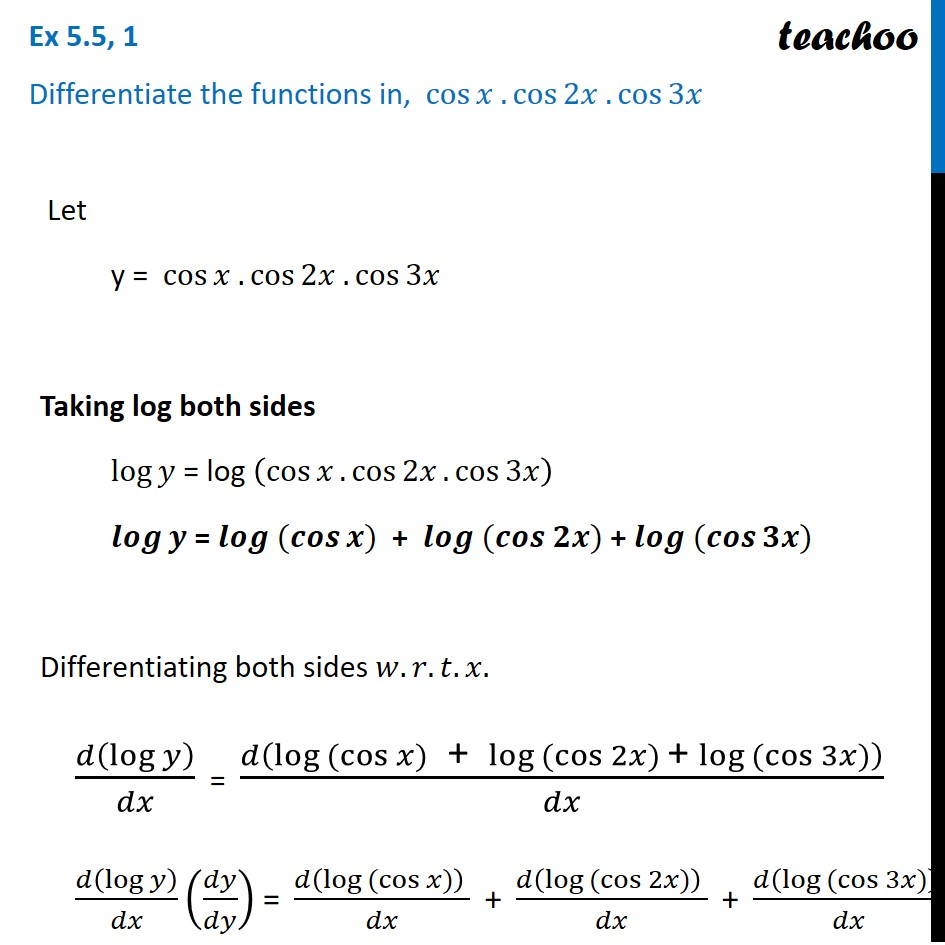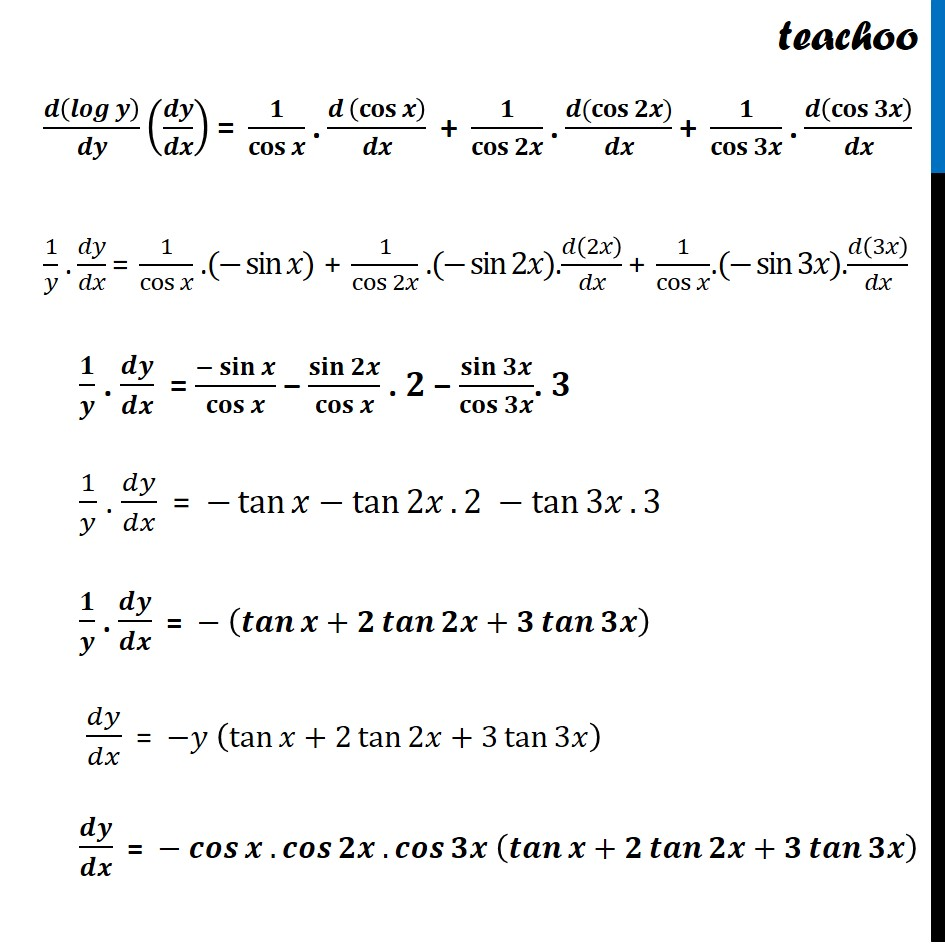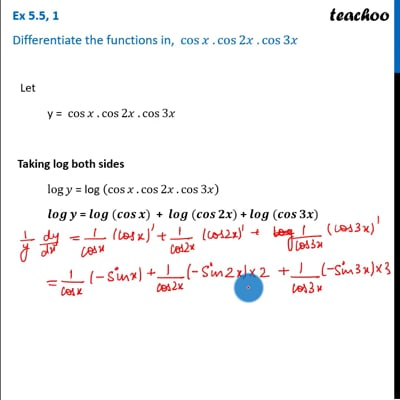Ex 5.5

Chapter 5 Class 12 Continuity and Differentiability
Serial order wiseThis video is only available for Teachoo black users

Solve all your doubts with Teachoo Black (new monthly pack available now!)

### Transcript

Ex 5.5, 1 Differentiate the functions in, cos⁡𝑥 . cos⁡2𝑥 . cos⁡3𝑥 Let y = cos⁡𝑥 . cos⁡2𝑥 . cos⁡3𝑥 Taking log both sides log⁡𝑦 = log (cos⁡𝑥.cos⁡2𝑥.cos⁡3𝑥 ) 𝒍𝒐𝒈⁡𝒚 = 𝒍𝒐𝒈 ⁡(𝒄𝒐𝒔⁡𝒙) + 𝒍𝒐𝒈 ⁡(𝒄𝒐𝒔 𝟐𝒙) + 𝒍𝒐𝒈 ⁡(𝒄𝒐𝒔⁡𝟑𝒙) Differentiating both sides 𝑤.𝑟.𝑡.𝑥. 𝑑(log⁡𝑦 )/𝑑𝑥 = 𝑑(log ⁡(cos⁡𝑥)" + " log ⁡(cos⁡2𝑥) "+ " log ⁡(cos⁡3𝑥))/𝑑𝑥 𝑑(log⁡𝑦 )/𝑑𝑥 (𝑑𝑦/𝑑𝑦) = (𝑑(log ⁡(cos⁡𝑥)) )/𝑑𝑥 + (𝑑(log ⁡(cos⁡2𝑥)) )/𝑑𝑥 + (𝑑(log ⁡(cos⁡3𝑥)) )/𝑑𝑥 𝒅(𝒍𝒐𝒈⁡𝒚 )/𝒅𝒚 (𝒅𝒚/𝒅𝒙) = 𝟏/𝐜𝐨𝐬⁡𝒙 . (𝒅 (𝐜𝐨𝐬⁡𝒙 ))/𝒅𝒙 + 𝟏/𝐜𝐨𝐬⁡𝟐𝒙 . (𝒅(𝐜𝐨𝐬⁡𝟐𝒙))/𝒅𝒙 + 𝟏/𝐜𝐨𝐬⁡𝟑𝒙 . 𝒅(𝐜𝐨𝐬⁡𝟑𝒙 )/𝒅𝒙 1/𝑦 . 𝑑𝑦/𝑑𝑥 = 1/cos⁡𝑥 .(− sin⁡𝑥) + 1/cos⁡2𝑥 .(− sin⁡2𝑥).𝑑(2𝑥)/𝑑𝑥 + 1/cos⁡𝑥 .(− sin⁡3𝑥).𝑑(3𝑥)/𝑑𝑥 𝟏/𝒚 . 𝒅𝒚/𝒅𝒙 = (−𝐬𝐢𝐧⁡𝒙)/𝐜𝐨𝐬⁡𝒙 − 𝐬𝐢𝐧⁡𝟐𝒙/𝐜𝐨𝐬⁡𝒙 . 𝟐 − 𝐬𝐢𝐧⁡𝟑𝒙/𝐜𝐨𝐬⁡𝟑𝒙 . 𝟑 1/𝑦 . 𝑑𝑦/𝑑𝑥 = −tan⁡𝑥−tan⁡2𝑥. 2 −tan⁡3𝑥. 3 𝟏/𝒚 . 𝒅𝒚/𝒅𝒙 = − (𝒕𝒂𝒏⁡𝒙+𝟐 𝒕𝒂𝒏⁡𝟐𝒙+𝟑 𝒕𝒂𝒏⁡𝟑𝒙 ) 𝑑𝑦/𝑑𝑥 = −𝑦 (tan⁡𝑥+2 tan⁡2𝑥+3 tan⁡3𝑥 ) 𝒅𝒚/𝒅𝒙 = − 𝒄𝒐𝒔⁡𝒙 . 𝒄𝒐𝒔⁡𝟐𝒙 . 𝒄𝒐𝒔⁡𝟑𝒙 (𝒕𝒂𝒏⁡𝒙+𝟐 𝒕𝒂𝒏⁡𝟐𝒙+𝟑 𝒕𝒂𝒏⁡𝟑𝒙 )